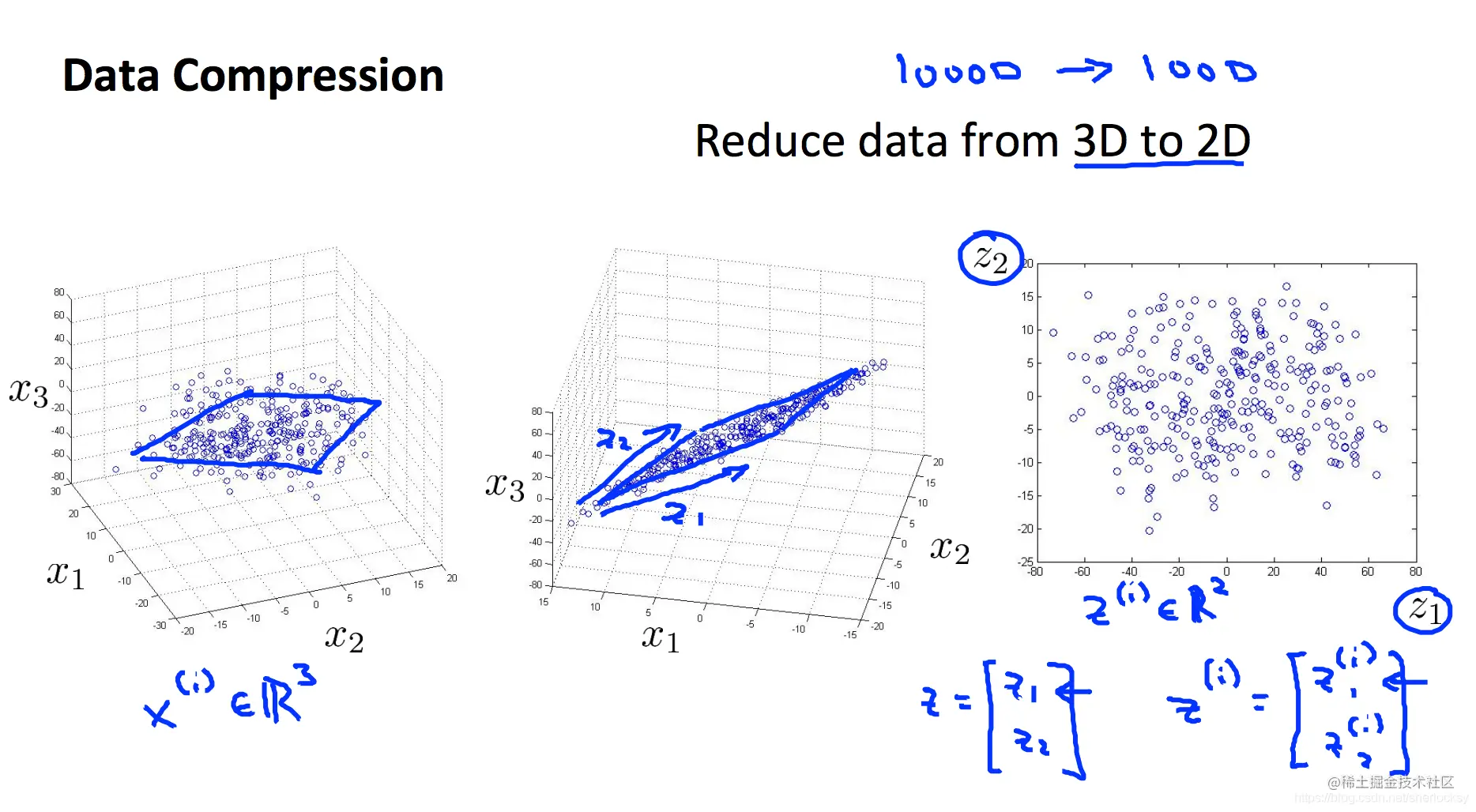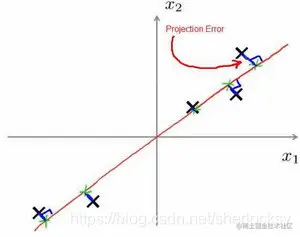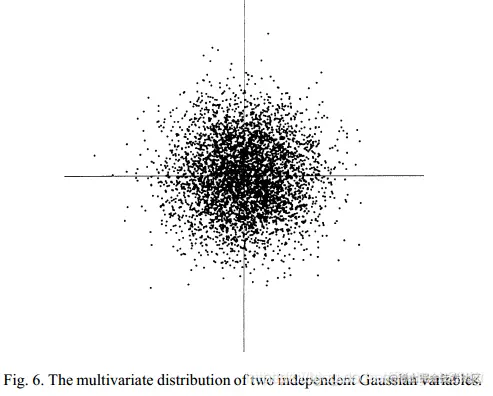# PCA and ICA for introduction to machine learning

2022-01-26 23:29:42

This is my participation 8 The fourth of the yuegengwen challenge 12 God , Check out the activity details ：8 Yuegengwen challenge

This article is the sixth in the notes series of Wu Enda's machine learning course , Mainly about the commonly used algorithms for data dimensionality reduction -PCA Principal component analysis algorithm , At the same time, extend another algorithm -ICA Independent component analysis .

# Principal component analysis PCA

Before learning principal component analysis , Let's first understand what dimensionality reduction is .

## What is dimension reduction

Refer to the definition of watermelon book , Dimension reduction That is, the original high-dimensional attribute space is transformed into a low-dimensional subspace through some mathematical transformation . In this subspace, the sample density is greatly increased , Distance calculation will also become easier .

In fact, dimensionality reduction is generally from high-dimensional projection to low-dimensional projection .

Such as below , about 3 D data , drop to 2 Dimension is to change the data from the original 3 Projection of dimensional space to 2 Dimensional plane , This enables dimensionality reduction .## PCA (Principal Component Analysis)

Principal component analysis is the most common dimensionality reduction algorithm .PCA Can extract main components from redundant features , Without much loss of model quality , Improved model training speed .

Projection error （Projection Error）The projection error is ： Project the data onto a vector passing through the origin （ The direction vector ）, The length of the perpendicular line between the eigenvector and the direction vector .

PCA The goal is , We want to find a direction vector （Vector direction）, Make the mean square error of this projection error as small as possible .

Obviously, this is an example of reducing to one dimension . that PCA The complete description of is ：

Want to n Dimensional data reduced to k dimension , The goal is to find such a set of vectors $u_1,u_2,u_3,...,u_k$ , The total projection error of all data projected on this set of vectors is minimized . In fact, this set of vectors should be orthogonal in the original space . And this k The new orthogonal feature of dimension is also called principal component , It's in the original n Based on the characteristics of dimension, we reconstruct k Whitman's sign .

### Algorithm flow

1. Mean normalization .

2. Calculate the covariance matrix ：

$\Sigma = \dfrac{1}{m} \sum\limits_{i=1}^{n}(x^{(i)})(x^{(i)})^T = \dfrac{1}{m} X^TX$

3. adopt Singular value decomposition Calculate the covariance matrix $\Sigma$ Of Eigenvector

$(U,S,V^T)=SVD(\Sigma)$

4. from $U$ Before you choose $k$ Vector , To obtain a $n\times k$ The matrix of dimensions , use $U_{reduce}$ Express . $k$ We want to take the data from $n$ Dimension reduction $k$ dimension .

5. Calculate the new eigenvector $z^{(i)}$:

$z^{(i)}=U^T_{reduce} * x^{(i)}$

obviously , The end result is naturally $k \times 1$ dimension .

in general , Want to get the principal component , First calculate the covariance matrix of the data matrix , Then the eigenvector of covariance matrix is obtained by singular value decomposition , Then select the one with the largest eigenvalue, that is, the one with the largest variance k A matrix of eigenvectors .

To sum up ,PCA As a dimension reduction method of unsupervised learning , It just needs eigenvalue decomposition , You can compress the data , Denoise . So it's widely used in real scenes .

# Independent component analysis ICA

above PCA It's a process of information extraction , Used to reduce the dimension of original data , And the next mentioned Independent component analysis namely ICA（Independent Component Analysis）, It is a process of information disambiguation .

## Problem introduction

ICA The premise is that the observed variable is a linear combination of several statistically independent components .

Let's start with the classic cocktail party （ocktail party problem） To discuss . This is the problem ： There is... In a room $n$ Someone is driving party, They can talk at the same time . In different corners of the room $n$ Sound receivers , Each receiver can simultaneously collect $n$ Overlapping sounds of personal voices . The distance between each receiver and each person is different , Therefore, the overlap of the sound received by each receiver is also different .party After the end , We get $m$ A sound sample , Each sample is at a specific time $i$, from $n$ A set of sound data collected by a receiver , How to start from this $m$ A sample set is separated $n$ Each speaker's own voice ？

Let's take a closer look at the problem description , use $s$ To represent the sound signal source emitted by everyone at all times , It's a $n\times m$ Matrix , Each line represents a person $m$ A sequence of sound signals at a time , common $n$ That's ok , namely $n$ personal .

$s=[s_1,s_2,...,s_n]^T$

$x$ Is sampled at every moment $n$ A linear combination of personal sound data . It's also a $n\times m$ Matrix . That is to say $m$ A moment , Then a total of $m$ Group samples , And each sample is $n$ Dimensional . The superscript here $i$ It means a certain moment , $x^{i}$ It's a component , It stands for $i$ Everyone who receives it all the time $n$ A linear combination of sound signals .

$x=\begin{bmatrix} |&|&\dots&|\\x^{(1)} & x^{(2)}&\dots&x^{(m)}\\|&|&\dots&|\end{bmatrix} \\ x^{(i)}=[x_1^{(i)},x_2^{(i)},...,x_n^{(i)}]^T$

So we have the following model ：

$x=As\\ \begin{bmatrix} |&|&\dots&|\\x^{(1)} & x^{(2)}&\dots&x^{(m)}\\|&|&\dots&|\end{bmatrix}=A\begin{bmatrix} s_1^{(1)}&s_1^{(2)}&\dots&s_1^{(m)}\\s_2^{(1)}&s_2^{(2)}&\dots&s_2^{(m)}\\\vdots&\vdots&\ddots&\vdots \\s_n^{(1)}&s_n^{(2)}&\dots&s_n^{(m)}\end{bmatrix}$

among , $A$ Is an unknown mixed matrix , obviously $A$ yes $n\times n$ Dimensional , And must be full rank .

Now the situation is $A、s$ It is unknown. , $x$ It is known. , We need to find a way $x$ To launch $s$ and $A$, This process is also called blind signal separation . That sounds a little amazing, Let's take a slow look at .

## Algorithm

Without losing generality , We can assume that both the mixed variable and the independent component have a zero mean ; If the raw data is not zero-mean, We can observe variables $x$ Standardization , Make the model zero mean model .

First , Let's make a change , Make $W=A^{-1}$, that $s^{(i)}=A^{-1}x^{(i)}=Wx^{(i)}$, $W$ It can also be expressed as $[w_1^T,w_2^T,...,w_n^T]^T$, So for each source signal component ：

$s_j^{(i)}=w^T_jx^{(i)}$

then , Let's assume that everyone's voice signal $s_j$ It's independent , And there is a probability density $p_s(s_j)$, So at a given moment $i$ The joint probability density of the source signal is ：

$p(s)=\prod_{j=1}^np_s(s_j)$

With $p(s)$, We want to get the probability of sampling the signal , How do you get it ？

Let's first recall the knowledge of probability theory , We know that the probability density can be derived from the cumulative distribution function , Let's find the cumulative distribution function first ：

$F_x(a)=P(x\le a)=P(As\le a)=P(s\le Wa)=F_s(Wa)$

Then find a derivative ：

$F'_x(a)=F'_s(Wa)=|W|p_s(Wa)=p_x(a)$

So there is ：

$p(x)=p_s(Wx)|W|=|W|\prod_{j=1}^np_s(w_j^Tx)$

### Based on maximum likelihood estimation

Likelihood function ：

$L(W)=p_s(Wx)|W|=|W|\prod_{j=1}^np_s(w_j^Tx)$

Given a training sample $x^{(i)}=(x^{(1)},x^{(2)},...,x^{(m})$ , Find the log likelihood ：

$lnL(W)= \sum_{i=1}^m\{ \sum_{j=1}^nln\;p_s(w^T_jx^{(i)})+ln|W|\}$

## ICA Classical assumptions and uncertainties

### Classic hypothesis

1. The components are independent of each other

This is a ICA One of the most basic and important principles of , It is very interesting that once this assumption is given , We can solve this model in a certain way . The explanation for this is , If any sequence of random variables （x1,x2,…,xn） They are statistically independent of each other , Then this means that we can't get random variables from the rest of the variables xj Any information .

2.ICA A very strong assumption ： Independent components obey non Gaussian distribution .

This is because , If the source signal is Gaussian , That is, the independent components are Gaussian , Then their joint probability distribution will be uniform , The density is completely symmetrical , Take the two-dimensional Gaussian distribution in the figure below as an example . You can see , Gaussian variable $(x_1,x_2)$ The distribution of any orthogonal transformation of has the same as $(x_1,x_2)$ Exactly the same distribution . Because random variables with Gaussian distribution have high-order cumulants of 0 Characteristics of , therefore ICA After decomposition A There may be an infinite number of .If the source signal is non Gaussian , that ICA The decomposition is unique . That's why one Generally, in standard independent component analysis, at most one component is allowed to obey Gaussian distribution .

3. Suppose the mixing matrix A It's the square

This is obvious , It's to make A reversible , Easy to calculate .

### uncertainty

Having the same statistical characteristics $x$ It may come from two different systems ：

$x=As=(A\alpha)(\alpha^{-1}s)$

It can also be understood that the sampled signal and noise signal are not identifiable nonidentifiable. The above formula shows that , After a linear transformation , Mixed matrix A and s It's not the only one , So we can't uniquely determine the original signal .

### ICA Uncertain factors

• The variance of independent components cannot be determined
• The order of independent components cannot be determined

# Summary

Whether it's PCA still ICA, You don't need to make specific assumptions about the distribution of the source signal .

If the observed signal is Gaussian , Then the source signal is also Gaussian , At this time PCA and ICA It is equivalent. .

majority ICA Our algorithm needs data preprocessing ： First use PCA obtain y, And then y Standardization of each component of （ That is, divide each component by its own standard deviation ） obtain z. After pretreatment z Satisfy the following properties ：

• z The components of are not related ;
• z The variance of each component of is 1.